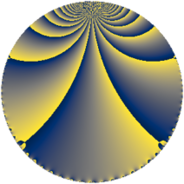# Properties

 Label 64.2.bLevel $64$ Weight $2$ Character orbit 64.b Rep. character $\chi_{64}(33,\cdot)$ Character field $\Q$ Dimension $2$ Newform subspaces $1$ Sturm bound $16$ Trace bound $0$

# Related objects

## Defining parameters

 Level: $$N$$ $$=$$ $$64 = 2^{6}$$ Weight: $$k$$ $$=$$ $$2$$ Character orbit: $$[\chi]$$ $$=$$ 64.b (of order $$2$$ and degree $$1$$) Character conductor: $$\operatorname{cond}(\chi)$$ $$=$$ $$8$$ Character field: $$\Q$$ Newform subspaces: $$1$$ Sturm bound: $$16$$ Trace bound: $$0$$

## Dimensions

The following table gives the dimensions of various subspaces of $$M_{2}(64, [\chi])$$.

Total New Old
Modular forms 14 2 12
Cusp forms 2 2 0
Eisenstein series 12 0 12

## Trace form

 $$2 q - 2 q^{9} + O(q^{10})$$ $$2 q - 2 q^{9} - 12 q^{17} + 10 q^{25} + 24 q^{33} - 12 q^{41} - 14 q^{49} - 8 q^{57} + 4 q^{73} - 22 q^{81} + 36 q^{89} + 20 q^{97} + O(q^{100})$$

## Decomposition of $$S_{2}^{\mathrm{new}}(64, [\chi])$$ into newform subspaces

Label Dim $A$ Field CM Traces $q$-expansion
$a_{2}$ $a_{3}$ $a_{5}$ $a_{7}$
64.2.b.a $2$ $0.511$ $$\Q(\sqrt{-1})$$ $$\Q(\sqrt{-2})$$ $$0$$ $$0$$ $$0$$ $$0$$ $$q+iq^{3}-q^{9}-3iq^{11}-6q^{17}+iq^{19}+\cdots$$